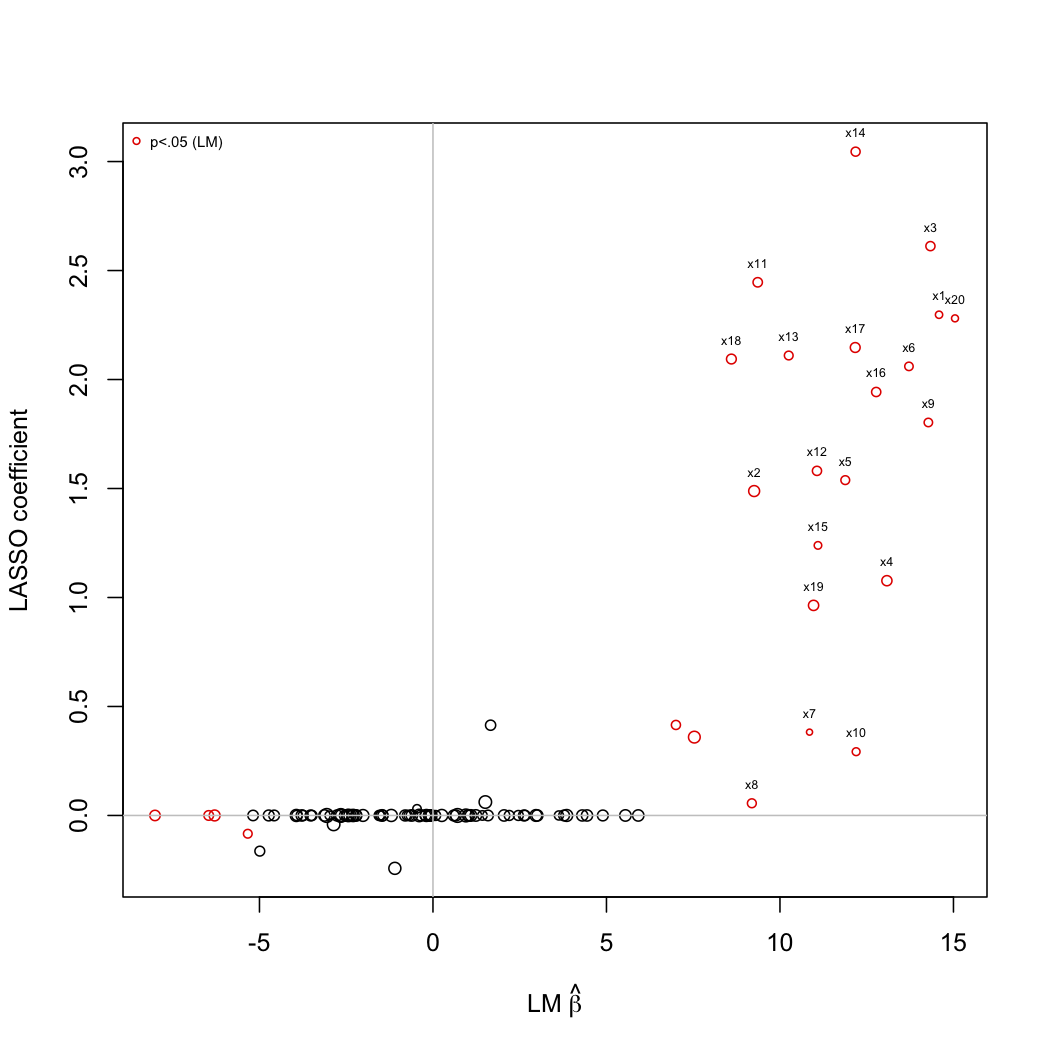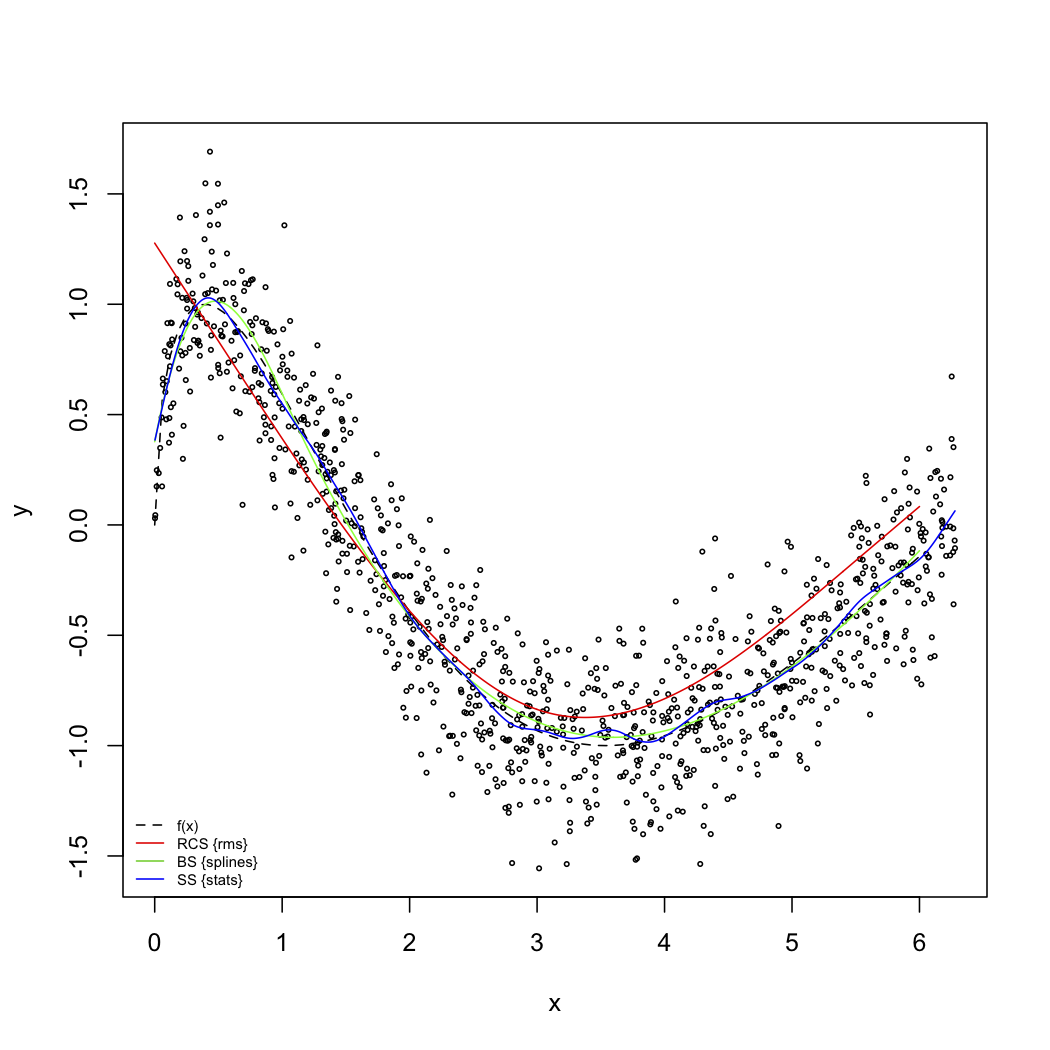# aliquot

## < a quantity that can be divided into another a whole number of time />

This is a recent book co-edited by Xiaochun Li and Ronghui Xu (Springer, 2009) about feature selection and model validation in the context of oncological studies. More precisely, the seven chapters cover (snip).

Here is a small simulation with LASSO penalization. The following picture depicts the coefficients estimated from simple univariate linear regression (x-axis) plotted against those estimated with LARS algorithm (LASSO mode). R code is shown below:

Basically, I simulated a set of p=100 predictors, with s=20 variables (symmetrically correlated at 0.3) associated to the Y outcome. Then, I would like to see if LASSO penalization allows to recover the true associated Xs. Clearly, it seems to works quite well, except for some predictors which would be judged significant through univariate testing (although in this case, correcting for multiple comparisons with, e.g., Bonferroni, would yield an alpha of 5e-04).The next figure illustrates some nice properties of splines fitting. The code used to produce this picture follows.